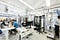# Setup & Installation

First, you’ll need to sign up for a free API key for Forest here. Once signed up, you’ll receive your API key within a few minutes via email.

`conda install -c rigetti pyquil`

# Import pyQuil

Now that you’ve installed pyQuil, open up your code editor, or spin up a Jupyter notebook, and create a new file named `quantum_dice.py`.

`from pyquil.quil import Programfrom pyquil.api import QVMConnectionfrom pyquil.gates import Hfrom functools import reduceqvm = QVMConnection()`
1. `QVMConnection` enables us to connect to a quantum virtual machine (QVM), a powerful 26-qubit simulator of a quantum computer. The QVM is great for testing and development, and towards the end of this post, I’ll show you how to replace the QVM with an API connection to the actual quantum computer itself.
2. `H` is the Hadamard gate. If you aren’t familiar with the Hadamard gate, don’t worry. For now, all we need to know is that it will apply a quantum state that helps us randomize the roll of the dice. I’ll return to this below in more detail.
3. `reduce` is not a part of pyQuil, but is a commonly used function in Python for performing loops and iterative computations. Specifically, this will help us format the final result of our rolled dice into an integer value between 1 and 8.

# Qubits, superposition, and quantum gates

Before moving on, let’s quickly introduce some quantum computing concepts.

# Creating the 8-sided dice with quantum bits

To illustrate how this works, let’s go back to our quantum dice program.

`dice = Program(H(0), H(1), H(2))`
• [0, 0, 1] The first two qubits are in state 0, the third in state 1
• [0, 1, 1] The first qubit is in state 0, the second and third in state 1
• [1, 1, 1] …
• [1, 1, 0]
• [1, 0, 1]
• [1, 0, 0]
• [0, 1, 0]

# Rolling the dice

Now that we have 8 equally random outcomes, we need to generate a single result, i.e. roll the dice.

`# Measure the qubits to get a result, i.e. roll the diceroll_dice = dice.measure_all()`
`print(roll_dice)# Output:H 0H 1H 2MEASURE 0 MEASURE 1 MEASURE 2 `
`# Execute the program by running it with the QVMresult = qvm.run(roll_dice)`
`dice_value = reduce(lambda x, y: 2*x + y, result, 0) + 1print("Your quantum dice roll returned:", dice_value)`

# Moving from the QVM to the QPU

To summarize, we’ve successfully simulated an 8-sided dice on a quantum computer using Rigetti’s quantum virtual machine.

`# Remove thisfrom pyquil.api import QVMConnection...qvm = QVMConnection()....result = qvm.run(roll_dice)`
`from pyquil.api import QPUConnection...qpu = QPUConnection('8Q-Agave')...result = qpu.run(roll_dice)`

We introduced how qubits, superposition, and quantum gates work in quantum programming, and built a quantum program in just 10 lines of code. We also demonstrated the exponential scaling power of quantum computers (N qubits = 2^N bits).A few members of the Rigetti team, and our quantum computers, in Berkeley, CA
• Quantum Computing Explained by David McMahon. A helpful supplement to the the book above, in particular for explaining linear algebra concepts in more detail.

Written by